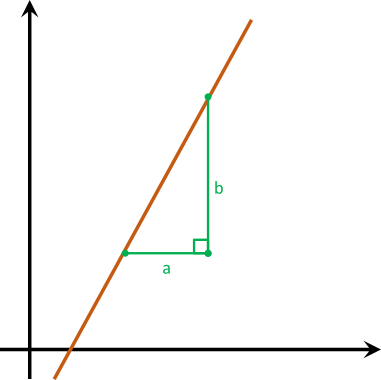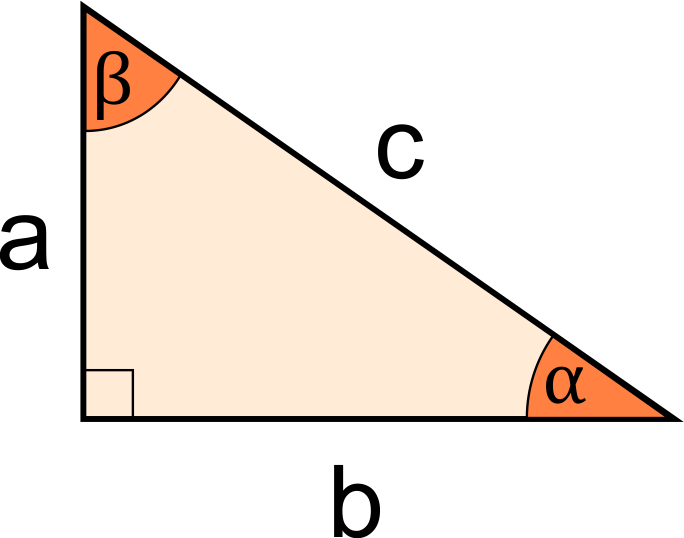# Triangle Slope Calculator

Created by Julia Żuławińska
Reviewed by Arturo Barrantes
Last updated: Jun 14, 2022

In the triangle slope calculator, you can determine a slope of a triangle or a line on a graph. Our calculator uses a simple slope of a triangle formula, where you only need to know two values - the lengths of the sides of the triangle drawn on a line. We can help you whether you want to get a quick answer to your problem or you're interested in understanding how to solve it on your own. In the text, we explain what a slope triangle is and how to find the slope of a triangle or a graphed line.

## What is a slope triangle?

When trying to find a slope of a line, you can use the slope triangle method. In that method, you draw two lines - horizontal and vertical - next to your line, which creates a triangle that can help you find the slope of the line.

In our triangle slope calculator, we use this formula to find the slope:

slope = a * rise / run

, where:

• a is equal to 1 if a line is rising and to -1 if the line is decreasing,
• rise is the length of the vertical side of the triangle, and
• run is the length of the horizontal side.## How to find the slope of a graphed line?

To find the slope of a graphed line, do the following steps:

1. Mark two points on the line.
2. From the point on the left, draw a horizontal line.
3. From the point on the right, draw a vertical line. Do you see a right triangle?
4. Measure how long the sections are from each point to the intersection of the created lines.
5. To find the slope, divide the rise (the length of the vertical segment) by the run (the length of the horizontal segment)
6. Is the line declining or rising? If the line rises, leave the slope value as it is - you have your result. If it's declining, remember that your slope should be negative, so put a minus sign in front of the slope value.

## How to find the slope of a triangle?

To be able to find the slope of a triangle, it needs to be a right triangle, and you also need to know one of the following:

• the length of the sides a and b,
• the angle α, or
• the angle β.If you know a and b, use this slope of a triangle formula to find the slope:

slope = rise/run = a/b

You can very quickly do those calculations in our triangle slope calculator.

🙋 A triangle's slope can change if you change the side the triangle lays on. So, in this formula, you should always divide the vertical side of the triangle by the horizontal side (rise over run).

How to find a triangle's slope if you have one of the two angles: α or β? If you know α you need to find the tangent of α:

slope = tan(α)

If you know β, the slope of the triangle would be equal to the cotangent of β:

slope = cot(β)

## Other useful tools like the triangle slope calculator

Since you already know how to calculate a triangle slope, you can take a look at other related calculators:

## FAQ

### How to graph a line with a 3:1 slope?

A 3:1 slope means a rise of 3 and a run of 1. If you want to graph a line with this slope, you need to:

1. Mark a point in any place.
2. Go 3 distances to the right.
3. Go 1 distance up.
4. Mark a new point.
5. Finally, draw the line between these two points.
Julia ŻuławińskaRise (a)
Run (b)
Are you looking for a slope of a triangle or line? If it's a line, from left to right, does it go up or down?
It's a triangle.
Result
Slope
People also viewed…

### Circumcenter of a triangle

With the circumcenter calculator you'll discover how to use the coordinates of a triangle's vertices to get the coordinates of the circumcenter.